Need Help?

Subscribe to Circuit

###### \${selected_topic_name}
• Notes

$\begin{array}{l}{\text { Use a Y-to- } \Delta \text { transformation to find the voltage }} \\ {v \text { in the circuit shown. }}\end{array}$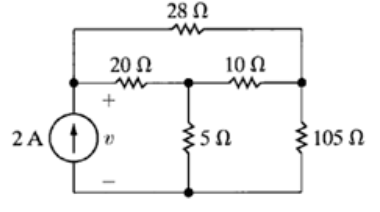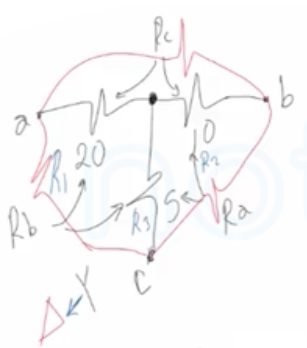$R_{a}=5+10+\frac{5 * 10}{20}=17.5 \Omega$

$R_{b}=20+5+\frac{20*5}{10}=35 \Omega$

$R_c=20+10+\frac{20*10}{5}=70 \Omega$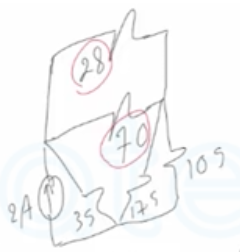$R_{eq}=\frac{28 * 70}{28+70}=20 \Omega$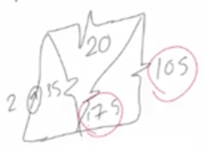$R_{eq}=\frac{17.5* 10{5}}{17.5+105}=15 \Omega$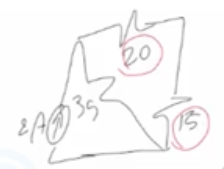$R_{eq}=20+15=35 \Omega$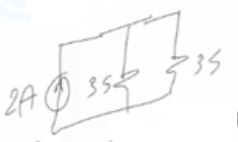$R_{e q}=\frac{35}{2}=17.5 \Omega$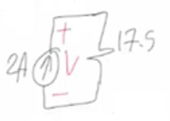$V=I R=2 * 17.5$

$V=35 V$